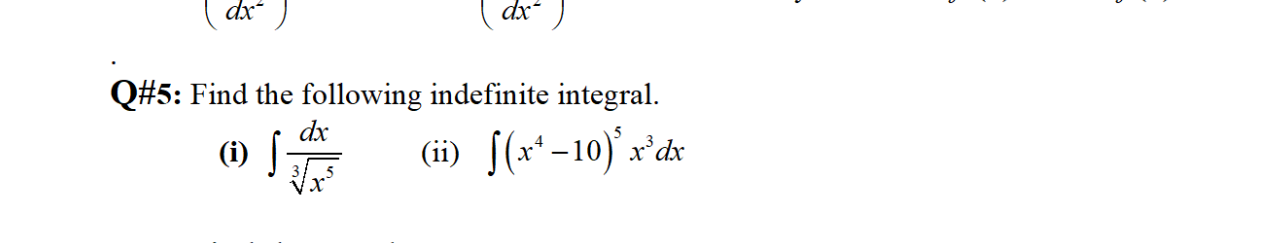Create an Account

Home / Questions / dx' ) dx- Q#5: Find the following indefinite integral. dx (i) s (ii) 5(x'-10)*x*dx

dx' ) dx- Q#5: Find the following indefinite integral. dx (i) s (ii) 5(x'-10)*x*dx

dx' ) dx- Q#5: Find the following indefinite integral. dx (i) s (ii) 5(x'-10)*x*dxMay 17 2021 View more View LessSubscribe To Get Solution